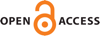Download this articleFor screen For printingRecent IssuesThe Journal About the Journal Editorial Board Editorial Interests Subscriptions Submission Guidelines Submission Page Policies for Authors Ethics Statement ISSN (electronic): 1472-2739 ISSN (print): 1472-2747 Author Index To Appear Other MSP JournalsThe $\mathrm{Sp}_{k,n}$–local stable homotopy category

### Drew Heard

Algebraic & Geometric Topology 23 (2023) 3655–3706##### Abstract

We study the category of $\left(K\left(k\right)\vee K\left(k+1\right)\vee \cdots \vee K\left(n\right)\right)$–local spectra, following a suggestion of Hovey and Strickland. When $k=0$, this is equivalent to the category of $E\left(n\right)$–local spectra, while for $k=n$, this is the category of $K\left(n\right)$–local spectra, both of which have been studied in detail by Hovey and Strickland. Based on their ideas, we classify the localizing and colocalizing subcategories, and give characterizations of compact and dualizable objects. We construct an Adams-type spectral sequence and show that when $p\gg n$ it collapses with a horizontal vanishing line above filtration degree ${n}^{2}+n-k$ at the ${E}_{2}$–page for the sphere spectrum. We then study the Picard group of $\left(K\left(k\right)\vee K\left(k+1\right)\vee \cdots \vee K\left(n\right)\right)$–local spectra, showing that this group is algebraic, in a suitable sense, when $p\gg n$. We also consider a version of Gross–Hopkins duality in this category. A key concept throughout is the use of descent.

##### Keywords
Morava $E$–theory, Morava $K$–theory, chromatic homotopy
##### Mathematical Subject Classification
Primary: 55P42, 55P60
Secondary: 55T15
##### Publication